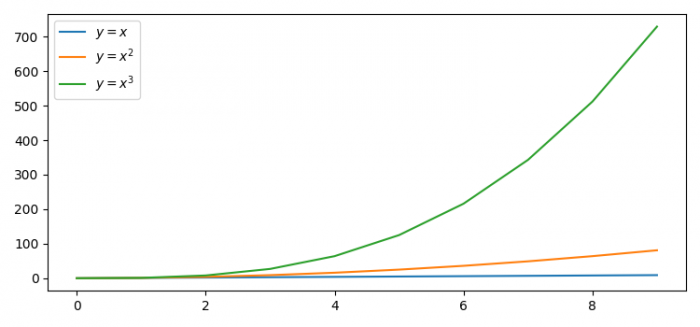# How can I get the color of the last figure in Matplotlib?

To get the color of the last figure, we can use get_color() method for every plot.

• Set the figure size and adjust the padding between and around the subplots.
• Create x and y data point using numpy.
• Plot (x, x), (x, x2) and (x, x3) using plot() method.
• Place a legend for every plot line.
• Get the color of each plot using get_color() method.
• To display the figure, use show() method.

## Example

import numpy as np
import matplotlib.pyplot as plt

plt.rcParams["figure.figsize"] = [7.50, 3.50]
plt.rcParams["figure.autolayout"] = True

x = np.arange(10)
y = np.arange(10)

p = plt.plot(x, y, x, y ** 2, x, y ** 3)

plt.legend([p, p, p], ["$y=x$", "$y=x^2$", "$y=x^3$"])

print("Color of the first plot: ", p.get_color())
print("Color of the second plot: ", p.get_color())
print("Color of the third plot: ", p.get_color())

plt.show()

## OutputAlong with the plots, you will get the following output printed on the console −

Color of the first plot: #1f77b4
Color of the second plot: #ff7f0e
Color of the third plot: #2ca02c